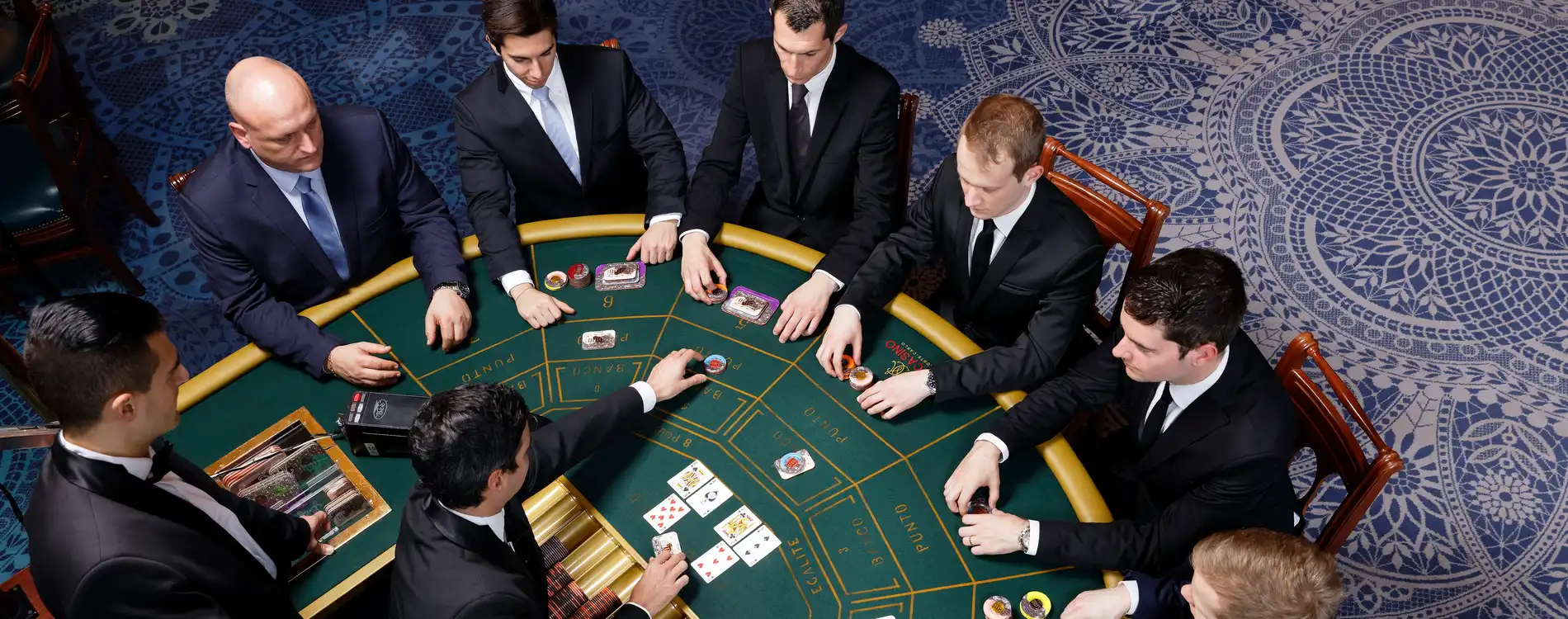Backstage
Spotlight

# Train your brain to stay sharp with our dealers

Published on April 20, 2020Updated on June 19, 2023

This week we’re going to take care of your brain, as it too needs exercise during this period of isolation.

To get you started, we’ve decided to provide some simple games and exercises that croupiers in the casinos  use during their training.

Croupiers—those mysterious, intractable arbiters of fast-paced casino games—need to be both shrewd psychologists and calculating machines in order to memorise numbers, stay concentrated and observe everything happening at the table. In order to process a flood of information and calculate quickly, they have to keep their brains sharp in a context where emotions are running high.

Between all the chips, bets and payouts, croupiers are basically top-level mathematicians. Here are a few exercises that will help you calculate quickly and get your grey matter working.

#  A GOOD MEMORY AND MENTAL CALCULATION ARE ESSENTIAL

The golden rule: a croupier must be unbeatable on the multiplication tables, he learns them by heart in ascending order but also in descending order! So, what if we tell you 9x8? You tense up, feel hot sweats… Don't panic! There are tips that we reveal to you thanks to the well-kept secrets of our croupiers.

Secret # 1: If you have to keep a few multipliers to shine in mental arithmetic, these are the multipliers of 3,6 and 12 and 2, 4 and 8.

Examples: 3 x 17 = 51 so 6 (3x2) x 17 will be double or 102.

12 x 17 = 204!

Multiples are very important because they allow easy deduction!

Secret n ° 2: To find all the results from the table of 9, follow the guide: List the numbers 0 through 9 under each other from top to bottom. Next, list the numbers from 0 to 9 above each other from bottom to top, facing the column formed. Previously. Unbelievable! the results of the table of 9 appear in order (09,18,27,36 etc ...)

So 9 x 8? 72 of course!Our brains need to be challenged as much as our bodies do.  Below you’ll find a few timed calculation exercises from the Ecole des Jeux that will help you stimulate your brain. So grab a pencil and paper, and get started! Note: you only have 90 seconds to complete this exercise

17 + 18 + 19 =                                     36 + 65 + 50 =

16 + (35-17) =                                     (25 - 9) + 18 =

(90/2) x 3 =                                         68 +105 +24 =

140 + 51 + 36 =                                  (700/4) x 11 =

(145 – 90) +15 =                                 (2500/4) x 11 =

(100/4) x 7 =                                       (150/2) x 3 =

(450/2) x 3 =                                       (900/4) x 5 =

50 +35 - 25 =                                        51 + 35 + 11=

(35 x 3) + 17 =                                     65 + 75 + 35 =

(2500/2) x 3 =                                     (210/6) x 7 =

Tick-tock: they might look easy, but these exercises are actually highly stimulating for your brain. See the answers HERE.